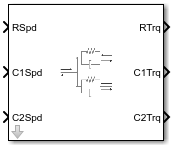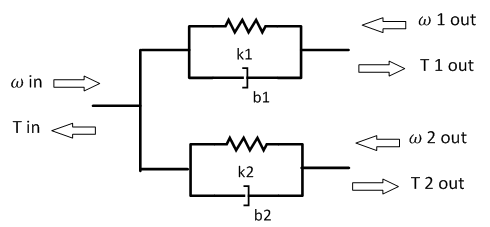# Split Torsional Compliance

Split torsional coupler

• Library:
• Powertrain Blockset / Drivetrain / Couplings

Vehicle Dynamics Blockset / Powertrain / Drivetrain / Couplings

•## Description

The Split Torsional Compliance block implements parallel spring-damper coupling between shafts. You can specify the type of coupling by selecting one of the Coupling Configuration parameters:

• `Shaft split` — Single input shaft coupled to two output shafts

• `Shaft merge` — Two input shafts coupled to a single output shaft

In fuel economy and emissions studies, you can use the Split Torsional Compliance block to model mechanical rotational compliance between common driveline elements such as motors, planetary gears, and clutches. For example, use the `Shaft split` configuration to couple a motor and two planetary gear sets. Use the `Shaft merge` configuration to couple a dual clutch transmission to an output shaft.

### Shaft Split

For the `Shaft split` configuration, the block implements this schematic and equations.`$\begin{array}{l}{T}_{in}=-\left({\omega }_{in}-{\omega }_{1out}\right){b}_{1}-\left({\omega }_{in}-{\omega }_{2out}\right){b}_{2}-{\theta }_{1}{k}_{1}-{\theta }_{2}{k}_{2}\\ {T}_{1out}=\left({\omega }_{in}-{\omega }_{1out}\right){b}_{1}+{\theta }_{1}{k}_{1}\\ {T}_{2out}=\left({\omega }_{in}-{\omega }_{2out}\right){b}_{2}+{\theta }_{2}{k}_{2}\\ \\ {\stackrel{˙}{\theta }}_{1}=\left({\omega }_{in}-{\omega }_{1out}\right)\\ {\stackrel{˙}{\theta }}_{2}=\left({\omega }_{in}-{\omega }_{2out}\right)\end{array}$`

To account for frequency-dependent damping, both damping terms incorporate a low-pass filter.

The equations use these variables.

 Tin Resulting applied input reaction torque ωin Input shaft rotational velocity T1out Resulting applied torque to first output shaft ω1out First output shaft rotational velocity T2out Resulting applied torque to second output shaft ω2out Second output shaft rotational velocity θ1, θ2 First, second shaft rotation, respectively b1, b2 First, second shaft viscous damping, respectively k1, k2 First, second shaft torsional stiffness, respectively

### Shaft Merge

For the `Shaft merge` configuration, the block implements this schematic and equations.`$\begin{array}{l}{T}_{out}=\left(-{\omega }_{out}+{\omega }_{1in}\right){b}_{1}+\left(-{\omega }_{out}+{\omega }_{2in}\right){b}_{2}+{\theta }_{1}{k}_{1}+{\theta }_{2}{k}_{2}\\ {T}_{1out}=\left({\omega }_{out}-{\omega }_{1in}\right){b}_{1}-{\theta }_{1}{k}_{1}\\ {T}_{2out}=\left({\omega }_{out}-{\omega }_{2in}\right){b}_{2}-{\theta }_{2}{k}_{2}\\ \\ {\stackrel{˙}{\theta }}_{1}=\left({\omega }_{1in}-{\omega }_{out}\right)\\ {\stackrel{˙}{\theta }}_{2}=\left({\omega }_{2in}-{\omega }_{out}\right)\end{array}$`

To account for frequency-dependent damping, both damping terms incorporate a low-pass filter.

The equations use these variables.

 Tout Resulting applied output torque ωout Output shaft rotational velocity T1in Resulting reaction torque to first input shaft ω1in First input shaft rotational velocity T2in Resulting reaction torque to second input shaft ω2in Second input shaft rotational velocity θ1, θ2 First, second shaft rotation, respectively b1, b2 First, second shaft viscous damping, respectively k1, k2 First, second shaft torsional stiffness, respectively

### Power Accounting

For the power accounting, the block implements these equations.

Bus Signal DescriptionVariableEquations

`PwrInfo`

`PwrTrnsfrd` — Power transferred between blocks

• Positive signals indicate flow into block

• Negative signals indicate flow out of block

`PwrR`

For the `Shaft split` configuration, mechanical power from input shaft

PTR

`PwrC1`

For the `Shaft split` configuration, mechanical power from first output shaft

PTC1

`PwrC2`

For the `Shaft split` configuration, mechanical power from second output shaft

PTC2

`PwrC`

For the `Shaft merge` configuration, mechanical power from output shaft

PTC

`PwrR1`

For the `Shaft merge` configuration, mechanical power from first input shaft

PTR1

`PwrR2`

For the `Shaft merge` configuration, mechanical power from second input shaft

PTR2

`PwrNotTrnsfrd` — Power crossing the block boundary, but not transferred

• Positive signals indicate an input

• Negative signals indicate a loss

`PwrDampLoss`Mechanical damping loss

Pd

${P}_{d}=-\left({b}_{1}{|{\stackrel{˙}{\theta }}_{1}|}^{2}+{b}_{2}{|{\stackrel{˙}{\theta }}_{2}|}^{2}\right)$

`PwrStored` — Stored energy rate of change

• Positive signals indicate an increase

• Negative signals indicate a decrease

`PwrStoredShft`

Rate change in spring energy

Ps

${P}_{s}=\left({k}_{1}{\theta }_{1}{\stackrel{˙}{\theta }}_{1}+{k}_{2}{\theta }_{2}{\stackrel{˙}{\theta }}_{2}\right)$

The equations use these variables.

 TR Shaft R torque TC Shaft C torque ωR Shaft R angular velocity ωC Shaft C angular velocity θ Coupled shaft rotation k Shaft torsional stiffness b Rotational viscous damping Pt Total mechanical power Pd Power loss due to damping Ps Rate change of stored spring energy

## Ports

### Input

expand all

Input shaft rotational velocity, ωin, in rad/s.

#### Dependencies

To enable this port, set both of these parameters:

• Port Configuration to `Simulink`

• Coupling Configuration to `Shaft split`

First output shaft rotational velocity, ω1out, in rad/s.

#### Dependencies

To enable this port, set both of these parameters:

• Port Configuration to `Simulink`

• Coupling Configuration to `Shaft split`

Second output shaft rotational velocity, ω2out, in rad/s.

#### Dependencies

To enable this port, set both of these parameters:

• Port Configuration to `Simulink`

• Coupling Configuration to `Shaft split`

Output shaft rotational velocity, ωout, in rad/s.

#### Dependencies

To enable this port, set both of these parameters:

• Port Configuration to `Simulink`

• Coupling Configuration to `Shaft merge`

First input shaft rotational velocity, ω1in, in rad/s.

#### Dependencies

To enable this port, set both of these parameters:

• Port Configuration to `Simulink`

• Coupling Configuration to `Shaft merge`

Second input shaft rotational velocity, ω2in, in rad/s.

#### Dependencies

To enable this port, set both of these parameters:

• Port Configuration to `Simulink`

• Coupling Configuration to `Shaft merge`

Input shaft angular velocity, ωin, in rad/s and torque, Tin, in N·m.

#### Dependencies

To enable this port, select:

• Port Configuration>`Two-way connection`

• Coupling Configuration>`Shaft split`

First input shaft angular velocity, ω1in, in rad/s and torque, T1in, in N·m.

#### Dependencies

To enable this port, select:

• Port Configuration>`Two-way connection`

• Coupling Configuration>`Shaft merge`

Second input shaft angular velocity, ω2in, in rad/s and torque, T2in, in N·m.

#### Dependencies

To enable this port, select:

• Port Configuration>`Two-way connection`

• Coupling Configuration>`Shaft merge`

### Output

expand all

If you set Coupling Configuration to `Shaft split`, the Info bus contains these signals.

SignalDescriptionVariableUnits
Trq

`R`

Input shaft torque

TinN·m

`C1`

First output shaft torque

T1outN·m

`C2`

Second output shaft torque

T2outN·m

`Damp`

`C1`

First output shaft damping torque

b1ω1out

N·m

`C2`

Second output shaft damping torque

b2ω2out

N·m

`Spring`

`C1`

First output shaft spring torque

k1θ1

N·m

`C2`

Second output shaft spring torque

k2θ2

N·m

Spd

`R`

Input shaft angular velocity

`C1`

First output shaft angular velocity

`C2`

Second output shaft angular velocity

`deltadot1`

Difference in input and first output shaft angular velocity

${\stackrel{˙}{\theta }}_{1}$

`deltadot2`

Difference in input and second output shaft angular velocity

${\stackrel{˙}{\theta }}_{2}$

PwrInfo

`PwrTrnsfrd`

`PwrR`

Mechanical power from input shaft

PTR

W
`PwrC1`

Mechanical power from first output shaft

PTC1

W
`PwrC2`

Mechanical power from second output shaft

PTC2W

`PwrNotTrnsfrd`

`PwrDampLoss`

Mechanical damping loss

Pd

W

`PwrStored`

`PwrStoredShft`

Rate change of stored internal torsional energy

Ps

W

If you set Coupling Configuration to `Shaft merge`, the Info bus contains these signals.

SignalDescriptionVariableUnits
Trq

`C`

Output shaft torque

ToutN·m

`R1`

First input shaft torque

T1inN·m

`R2`

Second input shaft torque

T2inN·m

`Damp`

`R1`

First input shaft damping torque

b1ω1in

N·m

`R2`

Second in shaft damping torque

b2ω2in

N·m

`Spring`

`R1`

First input shaft spring torque

k1θ1

N·m

`R2`

Second in shaft spring torque

k2θ2

N·m

Spd

`C`

Output shaft angular velocity

`R1`

First input shaft angular velocity

`R2`

Second input shaft angular velocity

`deltadot1`

Difference in first input and output shaft angular velocity

${\stackrel{˙}{\theta }}_{1}$

`deltadot2`

Difference in second input and output shaft angular velocity

${\stackrel{˙}{\theta }}_{2}$

PwrInfo

`PwrTrnsfrd`

`PwrC`

Mechanical power from output shaft

PTC

W
`PwrR1`

Mechanical power from first input shaft

PTR1

W
`PwrR2`

Mechanical power from second input shaft

PTR2W

`PwrNotTrnsfrd`

`PwrDampLoss`

Mechanical damping loss

Pd

W

`PwrStored`

`PwrStoredShft`

Rate change of stored internal torsional energy

Ps

W

#### Dependencies

To enable this port, select Output Info bus.

Input shaft torque, Tin, in N·m.

#### Dependencies

To enable this port, set both of these parameters:

• Port Configuration to `Simulink`

• Coupling Configuration to `Shaft split`

First output shaft torque, T1out, in N·m.

#### Dependencies

To enable this port, set both of these parameters:

• Port Configuration to `Simulink`

• Coupling Configuration to `Shaft split`

Second output shaft torque, T2out, in N·m.

#### Dependencies

To enable this port, set both of these parameters:

• Port Configuration to `Simulink`

• Coupling Configuration to `Shaft split`

Output shaft torque, Tout, in N·m.

#### Dependencies

To enable this port, set both of these parameters:

• Port Configuration to `Simulink`

• Coupling Configuration to `Shaft merge`

First input shaft torque, T1in, in N·m.

#### Dependencies

To enable this port, set both of these parameters:

• Port Configuration to `Simulink`

• Coupling Configuration to `Shaft merge`

Second input shaft torque, T2in, in N·m.

#### Dependencies

To enable this port, set both of these parameters:

• Port Configuration to `Simulink`

• Coupling Configuration to `Shaft merge`

First output shaft angular velocity, ω1out, in rad/s and torque, T1out, in N·m.

#### Dependencies

To enable this port, select:

• Port Configuration>`Two-way connection`

• Coupling Configuration>`Shaft split`

Second output shaft angular velocity, ω2out, in rad/s and torque, T2out, in N·m.

#### Dependencies

To enable this port, select:

• Port Configuration>`Two-way connection`

• Coupling Configuration>`Shaft split`

Output shaft angular velocity, ωout, in rad/s and torque, Tout, in N·m.

#### Dependencies

To enable this port, select:

• Port Configuration>`Two-way connection`

• Coupling Configuration>`Shaft merge`

## Parameters

expand all

Block Options

Specify the port configuration.

Specify the coupling type.

Select to create the `Info` output port.

Coupling 1

Torsional damping, b1, in N·m· s/rad.

Coupling 2

Torsional damping, b2, in N·m· s/rad.